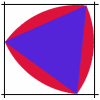#### You may also like### Marbles

I start with a red, a green and a blue marble. I can trade any of my marbles for two others, one of each colour. Can I end up with five more blue marbles than red after a number of such trades?### Rolling Triangle

The triangle ABC is equilateral. The arc AB has centre C, the arc BC has centre A and the arc CA has centre B. Explain how and why this shape can roll along between two parallel tracks.### Nine Colours

Can you use small coloured cubes to make a 3 by 3 by 3 cube so that each face of the bigger cube contains one of each colour?

# More Marbles

##### Age 11 to 14 Challenge Level:

Ling Xiang Ning from Singapore sent the following solution to the last two parts. Nice work Xiang Ning.

Suppose I start the marble trading with 4 marbles one of each colour red, blue, green and yellow. We can write the numbers of each colour in brackets, for example (1,2,3,4) stands for 1 red, 2 blue, 3 green and 4 yellow. For one of each colour we write (1,1,1,1) and after trading a yellow for one of each of the other three colours we have (2,2,2,0).

The question is can I trade marbles so that I will have exactly two marbles of each colour?

The total number of marbles at each stage is given by the sequence 4, 6, 8, 10, 12, ... whichever colours we trade. There is no way of getting 2 of each colour because I will have 8 marbles after 2 trades and whatever colours I have traded I always have an odd number of each colour at that stage.

The next part of the question asks can I have equal even numbers of each colour after a number of trades? The answer to this is also no, this is impossible, as we now show.

It is not possible to have equal even numbers of marbles of each colour after any number of trades. If we first trade a yellow for one of each of the other three colours we have (2,2,2,0). If you do a few trades, say for example, (1,1,1,1) -> (2,2,2,0) -> (1,3,3,1) -> (2,2,4,2) etc. you can see that the number of marbles of each colour turns from odd to even at each trade so you can only have an even number of each colour after an odd number of trades.

Each trade adds 2 to the total number of marble so after an odd number of trades (remember I have to have an odd number of trades to get an even number of each colour) I have 6, 10, 14, 18, 22, .... marbles altogether. After 2n+1 trades I have the 4 marbles I started with plus 2 times (2n+1) more which is 4 + 2(2n+1) = 4n+6 marbles.

We can now prove that it is impossible to get an equal even number of each colour by using an argument by contradiction. Suppose I were to have an even number, say 2k, of each colour at some stage, that is a total of 8k marbles which is divisible by 4. This is impossible because 4n + 6 is not divisible by 4.

Xiang Ning has answered the following questions:

What about ending up with 5 marbles of each colour? Is this possible and if so how many trades does it take? Is it possible to end up with the same odd number of each colour for any odd number?

I noticed that:

Take n as a odd number

n, n, n, n

--> n+1, n+1, n+1, n-1( after first trade)

--> n, n+2, n+2, n (after second trade)

--> n+1, n+1, n+3, n+1(after third trade)

--> n+2, n+2, n+2, n+2 (after fourth (2/2*4) trade, all numbers are odd)

...

--> n+4, n+4, n+4, n+4 (after 8(4/2*4) trades)

...

--> n+6, n+6, n+6, n+6 (after 12(6/2*4) trades)

...

we take 1 as n

so, 5 is n+4

4/2*4= 8

It is possible to end up with 5 marbles of each colour, and it takes 8 trades.

If we do a few trades, like (1,1,1,1) --> (2,2,2,0) --> (3,1,3,1) --> (2,2,4,2) --> (3,3,3,3)..., we can see that the number of marbles of each number turns from odd to even at each trade so you can only have an odd number of each colour after an even number of trades.

Each trade adds 2 to the total number of marbles so after an even number of trades (Remember I can only get an odd number of each colour after an even number of trades) I have 8, 12, 16, 20... marbles altogether. After 2n trades I have the 4 marbles I started with plus 2 times (2n) more which is 4 + 2(2n) = 4n + 4 marbles.

As 4n+4 is divisible by 4, it is possible to end up with the same odd number of each colour for any odd number.

So, it is possible to end up with the same odd number of each colour for any odd number.

Done by:

Name: Ling Xiang Ning, Allan

School: Tao Nan School

Country: Singapore